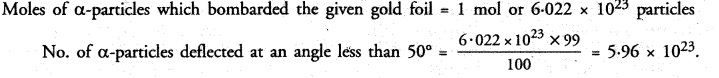## NCERT Exemplar Solutions for Class 9 Science Chapter 4 Structure of the Atom

These Solutions are part of NCERT Exemplar Solutions for Class 9 Science . Here we have given NCERT Exemplar Solutions for Class 9 Science Chapter 4 Structure of the Atom

Multiple Choice Questions

Question 1.
Which of the following correctly represents the electonic distribution in the Mg atom ?
(a) 3, 8, 1         (b) 2, 8, 2
(c) 1, 8, 3         (d) 8, 2, 2.
(b).

More Resources

Question 2.
Rutherfords alpha (a) particles scattering experiment resulted in to discovery of :
(a) Electron
(b) Proton
(c) Nucleus in the atom
(d) Atomic mass.
(c).

Question 3.
The number of electrons in an element X is 15 and the number of neutrons is 16. Which of the following is the correct representation of the element ?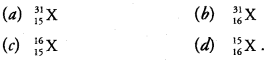(a) Mass no. = no. of electrons (protons) + no. of neutrons = 15 + 16 = 31.

Question 4.
Daltons atomic theory successfully explained :
(i) Law of conservation of mass
(ii) Law of constant composition
(iv) Law of multiple proportion
(a) (i), (ii) and (iii)         (b) (i), (ii) and (iv)
(c) (ii), (iii) and (iv)       (d) (i), (ii) and (iv).
(d) Except for the law of radioactivity, Dalton’s atomic theory explained all other laws which have been listed.

Question 5.
Which of the following statements about Rutherford’s model of atom are correct ?
(i) Considered the nucleus as positively charged
(ii) Established that the α-particles are four times as heavy as a hydrogen atom
(iii) Can be compared to solar system
(iv) Was in agreement with Thomson’s model
(a) (i) and (iii)         (b) (ii) and (iii)
(c) (i) and (iv)         (d) only (i).
(a) The statements (i) and (iii) are both correct.

Question 6.
Which of the following are true for an element ?
(i) Atomic number = number of protons + number of electrons
(ii) Mass number = number of protons + number of neutrons
(iii) Atomic Mass = number of protons + number of neutrons
(iv) Atomic number = number of protons = number of electrons
(a) (i) and (ii)           (b) (i) and (iii)
(c) (ii) and (iii)         (d) (ii) and (iv).
(d) The statements (it) and (iv) are both correct.

Question 7.
In the Thomson’s model of atom, which of the following statements are correct ?
(i) The mass of the atom is assumed to be uniformly distributed over the atom
(ii) The positive charge is assumed to be uniformly distributed over the atom
(iii) The electrons are uniformly distributed in the positively charged sphere
(iv) The electrons attract each other to stabilise the atom
(a) (i), (ii) and (iii)             (b) (i) and (iii)
(c) (i) and (iv)                   (d) (i), (iii) and (iv).
(a) Except for the statements (iv), all other statements are correct.

Question 8.
Rutherford’s α-particle scattering experiment showed that
(i) electrons have negative charge
(ii) the mass and positive charge of the atom is concentrated in the nucleus
(iii) neutron exists in the nucleus
(iv) most of the space in atom is empty Which of the above statements are correct ?
(a) (i) and (iii)        (b) (ii) and (iv)
(c) (i) and (iv)        (d) (iii) and (iv).
(b) The statements (ii) and (iv) are correct.

Question 9.
The ion of an element has 3 positive charges. Mass , number of the atom is 27 and the number of neutrons is 14. What is the number of electrons in the ion ?
(a) 13      (b) 10
(c) 14      (d) 16.
(b) The ion has 13 protons (27-14) and 10 electrons. It is Al3+ ion.

Question 10.
Identify the Mg2+ ion from the given figure where n and p represent the number of neutrons and protons respectively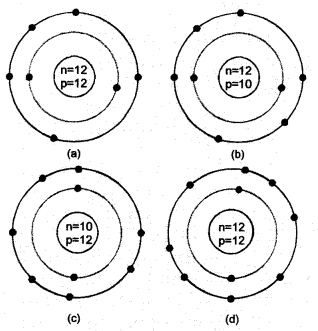(d) Since the ion has 10 electrons, it is Mg2+ ion.

Question 11.
In a sample of methyl ethanoate (CH3COOCH3) the two oxygen atoms have the same number of electrons but different number of neutrons. Which of the following is the correct reason for it ?
(a) One of the oxygen atoms has gained electrons
(b) One of the oxygen atoms has gained two neutrons
(c) The two oxygen atoms are isotopes
(d) The two oxygen atoms are isobars.
(c) The two oxygen atoms are the isotopes. These are the different atoms of the same element which have same number of electrons (or atomic number) but different number of neutrons.

Question 12.
Elements with valency 1 are :
(a) always metals
(b) always metalloids
(c) either metals or non-metals
(d) always non-metals.
(c) Elements with valency 1 may be metals (+1 valency) or non-metals (-1 valency)

Question 13.
The first model of an atom was given by :
(a) N. Bohr
(b) E. Goldstein
(c) Rutherford
(d) J.J. Thomson.
(d).

Question 14.
An atom with 3 protons and 4 neutrons will have a valency of :
(a) 3         (b) 7
(c) 1         (d) 4.
(c) The atom has also 3 electrons with electronic configuration 2, 1. It has valency of 1.

Question 15.
The electronic distribution in an aluminium atom is :
(a) 2, 8, 3         (b) 2, 8, 2
(c) 8, 2, 3         (d) 2, 3, 8.
(d).

Question 16.
Which of the following do not represent Bohr’s model of an atom correctly ?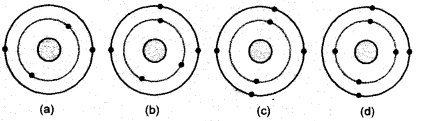(a) (i) and (ii)           (b) (ii) and (iii)
(c) (ii) and (iv)          (d) (i) and (iv).
(c) The first shell (K) cannot have more than two electrons in it.

Question 17.
Which of the following statements is always correct ?
(a) An atom has equal number of electrons and protons.
(b) An atom has equal number of electrons and neutrons.
(c) An atom has equal number of protons and neutrons.
(d) An atom has equal number of electrons, protons and neutrons.
(a) The number of electrons or protons in an atom are always equal.

Question 18.
Atomic models have been improved over the years. Arrange the following atomic models in the order of their chronological order :
(i) Rutherford’s atomic model
(ii) Thomson’s atomic model
(iii) Bohr’s atomic model
(a) (i), (ii) and (iii)
(b) (ii), (iii) and (i)
(c) (ii), (i) and (iii)
(d) (iii), (ii) and (i).
(c). It is the correct order.

Question 19.
An element has one proton, one electron and no neutron. Name the element. How will you represent it ?
The element is called protium or hydrogen (H). It can be represented as 11H.

Question 20.
Write any two observations to show that atoms are divisible.
The discovery of electrons and protons led to the opinion that the atoms are divisible. Even neutrons were also discovered at a later stage.

Question 21.
The atomic number (Z) of the element (Cl) is 17. Its electronic distribution is 2, 8, 7. It has valency (8 – 7) = 1 which is the same for both the species, which act as isotopes.

Question 22.
Why did Rutherford select a gold foil in his α-scattering experiment ?
Gold is a highly malleable. It could be converted into very fine foils or leaves. Moreover, the nucleus of the element is very heavy. It could not be displaced by the impact of fast moving α-particles.

Question 23.
Find out the valency of the atoms represented by the Fig. (a) and (b).Answer:
(a) The electronic configuration of the atom is 2, 8, 8. It has completely filled K, L, M shells. Its valency is zero. The atom belongs to the element Argon (Ar).
(b) The electronic configuration of the atom is 2, 7. It has seven electrons in the valence shell. Its valency is (8 – 7) equal to one. The atom belongs to the element fluorine (F).

Question 24.
One electron is present in the outermost shell of the atom of an element X. What would be the nature and value of charge on the ion formed if this electron is removed from the outermost shell ?
Since the atom of the element X has lost one electron from the outermost shell, it has +1 unit charge.

Question 25.
Write down the electron distribution of chlorine atom. How many electrons are there in the L shell ? (Atomic number of chlorine is 17).
The electronic distribution in chlorine (Z = 17) is K(2), L(8) and M(7). The L-shell has eight electrons.

Question 26.
In the atom of an element X, 6 electrons are present in the outermost shell. If it acquires noble gas configuration by accepting requisite number of electrons, then what would be the charge on the ion so formed ?
With six (6) electrons in the outermost shell, the atom of the element needs two (2) electrons more to have eight (8) electrons in the outermost shell. The ion (x2-) has -2 units charge.

Question 27.
What information do you get from the following electronic distribution about the atomic number, mass number and valency of atoms X, Y and Z ? Give your answer in a tabular form.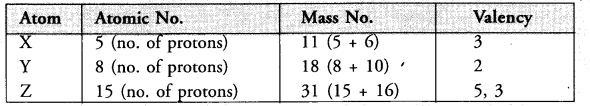• In atom X, valency is equal to number of valence electrons (2, 3) = 3
• Atom Y has electronic configuration 2, 6. Valency is eight – No. of valence electrons (8-6)
• In atom Z, valency is equal to number of valence electrons (2, 8, 5), or eight – no. of valence electrons
(8 – 5) = 3.

Question 28.
In response to a question, a student stated that in an atom, the number of protons is greater than the number of neutrons, which in turn is greater than the number of electrons. Do you agree with the statement ? Justify your answer.
The statement is wrong. In an atom, number of protons and electrons are always equal. These can be different only in an ion (positive or negative).

Question 29.
Calculate the number of neutrons present in the nucleus of an element 3115X which is represented as .
Mass no. of the element (A) = 31
Atomic no. of the element (Z) =15
No. of neutrons (n) = A – Z = 31 – 15 = 16.

Question 30.
Match the names of the Scientists given in column A with their contributions towards the understanding of the atomic structure
as given in column B.(a) — (iii) ;
(b) — (iv);
(c) — (i) ;
(d) — (ii)
(e) — (vi) ;
(f) — (vii) ;
(g) — (v).

Question 31.
The atomic numbers of calcium and argon are 20 and 18 respectively, but the mass number of both these elements is 40. What is the name given to such a pair of elements ?
The elements having same mass number but different atomic numbers are known as isobars.

Question 32.
Complete the following table on the basis of information available in the symbols given below :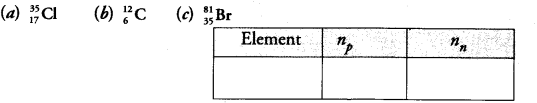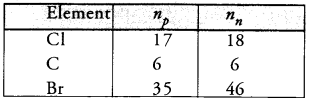Question 33.
Helium atom has 2 electrons in its valence shell but its valency is not 2. Explain.
Helium (He) atom with atomic number (Z) equal to two has only one shell (K-shell). It can have a maximum of two electrons which it has. Therefore, the valence shell is complete and valency of the atom is zero.

Question 34.
Fill in the blanks in the following statements :
(a) Rutherfords α-particle scattering experiment led to the discovery of the .
(b) Isotopes have same but different
(c) Neon and chlorine have atomic numbers 10 and 17 respectively. Their valencies will be
and respectively.
(d) The electronic configuration of silicon is and that of sulphur is
(a) nucleus
(b) atomic numbers, mass numbers
(c) zero and one
(d) 2, 8, 4 and 2, 8, 6.

Question 35.
An element X has a mass number 4 and atomic number 2. Write the valency of this element.
The element X with mass number 4 and atomic number 2 is helium (He). It has 2 electrons (maximum possible) in its only shell which is K-shell. Therefore, the valency of the element is zero.

Question 36.
Why do Helium, Neon and Argon have zero valency ?
All the three elements have completely filled valence shells, according to Bohr Bury scheme. Therefore, their valencies are zero.

Question 37.
The ratio of the radii of hydrogen atom and its nucleus is 105. Assuming the atom and the nucleus to be spherical,
(i) what will be the ratio of their sizes ?
(ii) If atom is represented by planet earth ‘Re’ = 6.4 x 106 m, estimate the radius of the nucleus.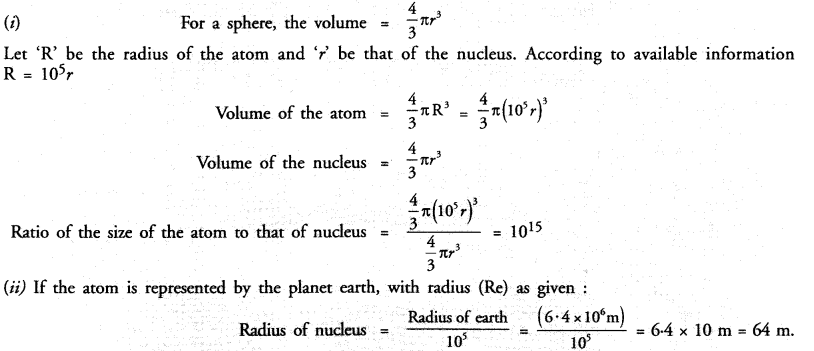Question 38.
Enlist the conclusions drawn by Rutherford from his α-ray scattering experiment.
He made the following conclusions from the experiment.

1. As most of the alpha particles passed through undeflected, this means that they did not come across any obstruction in their path. Thus, most of the space in an atom is expected to be empty.
2. As a few alpha particles suffered minor deflections and a very few major deflections, this means that these must have met with some obstructions in their path.
3. This obstruction must be :
1. Very small : Only a few particles were obstructed by it.
2. Massive : Each alpha particle has 4 u mass and is quite heavy. It could easily pass through a light obstruction by pushing it aside.

Question 39.
In what way is the Rutherfords atomic model different from Thomsons atomic model ?
According to Thomsons model, an atom may be regarded as a positively charged sphere containing protons in which the negatively charged protons are supposed to be studied or embedded. He gave no clue about the nucleus and extranuclear portion. This was given for the first time by Rutherfords model atom with the help of α-ray scattering experiment.

Question 40.
What were the drawbacks of Rutherfords model of an atom ?
There are infact, two main drawbacks of Rutherford Model atom

1. Rutherford model of atom could not explain the stability of the atom.
2. Rutherford model of atom could not explain as to how the electrons are distributed in the extra nuclear portion in an atom.

Question 41.
What are the postulates of Bohr’s model of an atom ?
The main postulates of the theory are listed :

1. In the extra nuclear portion of an atom, the electrons revolve in well defined circular paths known as orbits.
2. These circular orbits are also known as energy levels or energy shells.
3. These have been designated as K, L, M, N, O, … (or as 1, 2, 3, 4, 5, …) based on the energy present.
4. The order of the energy of these energy shells is :
K<L<M<N<0 <…. or 1< 2< 3 < 4<5 <….
5. While revolving in an orbit, the electron is not in a position to either lose or gain energy. In other words, its energy remains stationary. Therefore, these energy states for the electrons are also known as stationary states.

Question 42.
Show diagramatically the electronic distribution in a sodium atom and a sodium ion and also give their atomic number.
The atomic number of sodium (Na) is 11. The electronic distribution in the atom is K(2) L(8) and L(1). Sodium
ion (Na+) is formed by removal of one electron from the atom. Its electronic distribution is K(2) and L(8). This means that Na+ ion has the elecronic configuration of Ne atom which is inert gas atom. The electronic distribution of the atom and ion are shown as follows :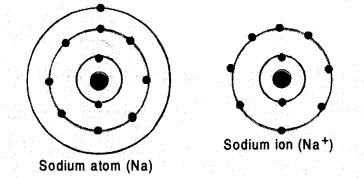Question 43.
In the Gold foil experiment of Geiger and Marsden, that paved the way for Rutherfords model of an atom, 1.00% of the α-particles were found to deflect at angles > 50°. If one mole of α-particles were bombarded on the gold foil, compute the number of α-particles that would deflect at angles less than 50°.